## ML Aggarwal Class 7 Solutions for ICSE Maths Chapter 12 Congruence of Triangles Ex 12.2

Question 1.
You want to establish ΔDEF = ΔMNP, using ASA rule of congruence. You are given that ∠D = ∠M and ∠F = ∠P. What additional information is needed to establish the congruence?
Solution:
In ΔDEF and ΔMNP
∠D = ∠M (Given)
∠F = ∠P (Given)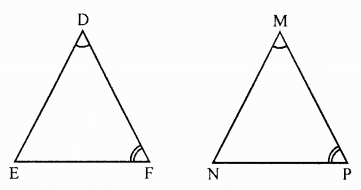For using ASA rule, we need
DF = MP (Included side)
Then ΔDEF = ΔMNP

Question 2.
In the given figure, two triangles are congruent. The corresponding parts are marked. We can write ΔRAT =?Solution:
In the given figure,
ΔART and ΔNOW
AT = NO (Given)
∠A = ∠O (Given)
∠T = ∠N (Given)
ΔART = ΔWON (ASA criterion)

Question 3.
If ΔABC and ΔPQR are to be congruent, name one additional pair of corresponding parts. What criterion did you use?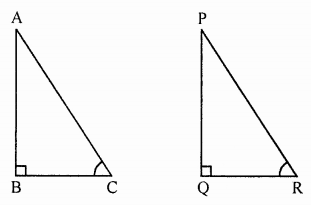Solution:
In ΔABC and ΔPQR
∠B = ∠Q
∠C = ∠Q
Now we need BC = QR
ΔABC = ΔPQR (ASA criterion)

Question 4.
Given below are measurements of some parts of two triangles. Examine whether the two triangles are congruent or not, by ASA congruence rule. In the case of congruence, write its in symbolic form.

 ΔDEF ΔPQR (i) ∠D = 60°, ∠F = 80°, DF = 5 cm (i) ∠Q = 60°, ∠R = 80°, QR = 5 cm (ii) ∠D = 60°, ∠F = 80°, DF = 6 cm (ii) ∠Q = 60°, ∠R = 80°, QR = 5 cm (iii) ∠E = 80°, ∠F = 30°, EF = 5 cm (iii) ∠P = 80°, PQ = 5 cm, ∠R = 30°

Solution:
In ΔDEF and ΔPQR
(i) ∠D = 60°, ∠F = 80°, DF = 5 cm
∠Q = 60°, ∠R = 80°, QR = 5 cm
∠D = ∠Q (Each 60°)
∠F = ∠R (Each 80°)
Included side DF = QR
ΔDEF = ΔQPR (ASA criterion)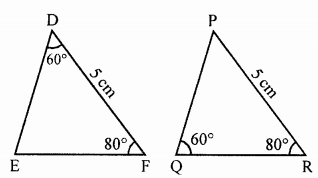(ii) In ΔDEF and ΔPQR
∠D = 60°, ∠F = 80°, DF = 6 cm
∠Q = 60°, ∠R = 80° and QP = 6 cm
Here, ∠D = ∠Q (Each 80°)
∠F = ∠R (Each 80°)
But included side DF ≠ QR
ΔDEF and ΔPQR are not congruent.(iii) In ΔDEF and ΔPQR
∠E = 80°, ∠F = 30°, EF = 5 cm
∠P = 80°, PQ = 5 cm, ∠R = 30°
Here, ∠E = ∠P (Each = 80°)
∠F = ∠R (Each = 30°)
But inlcuded sides are not equal.
ΔDEF and ΔPQR are not congruent.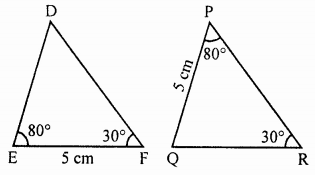Question 5.
In the adjoining figure, measures of some parts are indicated.
(i) State three pairs of equal parts in triangles ABC and ABD.
(ii) Is ΔABC = ΔBAD? Give reasons.
(iii) Is BC = AD? Why?Solution:
In the given figure,
∠DAC = 45°, ∠CAB = 30°, ∠CBD = 45° and ∠DBA = 30°
∠DAC + ∠CAB = 45° + 30° = 75°
and ∠CBD + ∠DBA = 45° + 30° = 75°
∠DAB = ∠CBA
Now in ΔABC and ΔDAB
AB = AB (Common)
∠CBA = ∠DAB (Proved)
∠CAB = ∠DBA (Each = 30°)
ΔABC = ΔDAB (ASA criterion)

Question 6.
In the adjoining figure, ray AZ bisects ∠DAB as well as ∠DCB.
(i) State the three pairs of equal parts in triangles BAC and DAC.
(ii) Is ΔBAC = ΔDAC? Give reasons.
(iii) Is CD = CB? Give reasons.Solution:
In the given figure
∠DAC = ∠BAC
∠DCA = ∠BCA
Now in ΔBAC and ΔDAC
AC = AC (Common)
∠BAC = ∠DAC (Given)
∠BCA = ∠DCA (Given)
ΔBAC = ΔDAC (ASA criterion)
Yes, CD = CB (c.p.c.t.)

Question 7.
Explain why ΔABC = ΔFED?Solution:
In ΔABC and ΔFED
BC = DE
∠B = ∠E (Each = 90°)
∠A = ∠F
∠C = 90° – ∠A and ∠D = 90° – ∠F
But ∠A = ∠F (Given)
∠C = ∠D
Now in ΔABC and ΔDEF
BC = DE (Given)
∠B = ∠E (Given 90°)
∠C = ∠D (Proved)
ΔABC = ΔDEF (ASA criterion)

Question 8.
Given below are the measurements of some parts of triangles. Examine whether the two triangles are congruent or not, using RHS congruence rule. In the case of congruent triangles, write the result in symbolic form:

 ΔABC ΔPQR (i) ∠B = 90°, AC = 8 cm, AB = 3 cm (i) ∠P = 90°, PR = 3 cm, QR = 8 cm (ii) ∠A = 90°, AC = 5 cm, BC = 9 cm (ii) ∠Q = 90°, PR = 8 cm, PQ = 5 cm

Solution:
We are given the measurement of some parts of the triangles.
We have to examine whether the two triangles are congruent
or not using RHS congruency rule.
In ΔABC and ΔPQR
(i) ∠B = 90°, AC = 8 cm, AB = 3 cm
∠P = 90°, PR = 3 cm, QR = 8 cm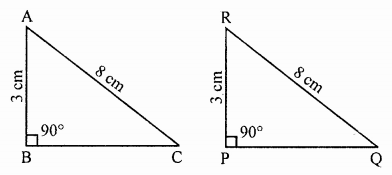We see that in two Δs ABC and RPQ
∠B = ∠P (Each = 90°)
Side AB = RP (Each = 3 cm)
Hypotenuse AC = RQ
ΔABC = ΔRPQ (RHS criterion)
(ii) In ΔABC and ΔPQR∠A = ∠Q (Each = 90°)
Side AC = QP (Each = 5 cm)
But hypotenuse BC and PR are not equal to each other.
Triangles are not congruent.

Question 9.
In the given figure, measurements of some parts are given.
(i) State the three pairs of equal parts in ΔPQS and ΔPRS.
(ii) Is ΔPQS = ΔPRS? Give reasons.
(iii) Is S mid-point of $$\bar { QR }$$ ? Why?Solution:
In the given figure,
PQ = 3 cm, PR = 3 cm
PS ⊥ QR
(i) Now in right ΔPQS and ΔPRS right angles at S. (∵ PS ⊥ QR)
side PS = PS (Common)
Hypotenuse PQ = PR (Each = 3 cm)
(ii) ΔPQS = ΔPRS (RHS criterion)
(iii) QS = SR (c.p.c.t.)
S is the mid point of QR

Question 10.
In the given figure, O is mid-point of $$\bar { AB }$$ and ∠A = ∠B. Show that ΔAOC = ΔBOD.Solution:
In the given figure,
O is the mid-point of AB
AO = OB
Now in ΔAOC and ΔBOD
AO = OB (∵ O is mid-point of AB)
∠A = ∠B (Given)
∠AOC = ∠BOD (Vertically opposite angles)
ΔAOC = ΔBOD (ASA criterion)